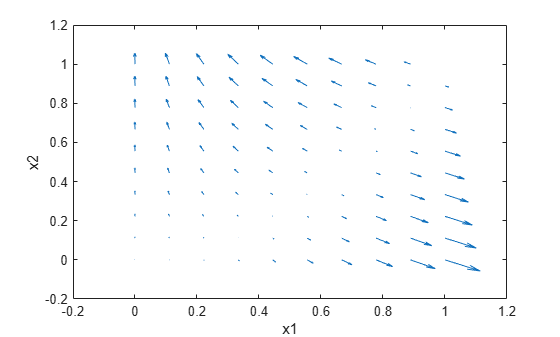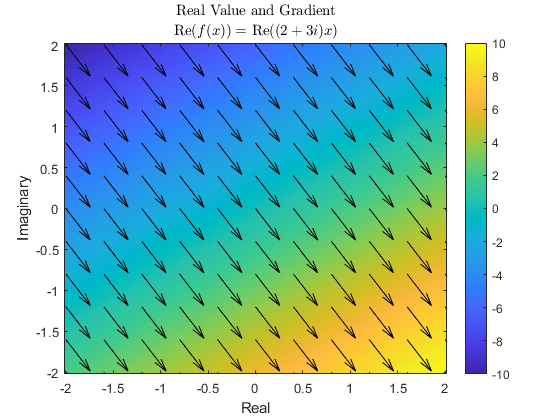Compute gradients for custom training loops using automatic differentiation

## Syntax

``[dydx1,...,dydxk] = dlgradient(y,x1,...,xk)``
``[dydx1,...,dydxk] = dlgradient(y,x1,...,xk,Name,Value)``

## Description

Use `dlgradient` to compute derivatives using automatic differentiation for custom training loops.

Tip

For most deep learning tasks, you can use a pretrained network and adapt it to your own data. For an example showing how to use transfer learning to retrain a convolutional neural network to classify a new set of images, see Train Deep Learning Network to Classify New Images. Alternatively, you can create and train networks from scratch using `layerGraph` objects with the `trainNetwork` and `trainingOptions` functions.

If the `trainingOptions` function does not provide the training options that you need for your task, then you can create a custom training loop using automatic differentiation. To learn more, see Define Deep Learning Network for Custom Training Loops.

example

````[dydx1,...,dydxk] = dlgradient(y,x1,...,xk)` returns the gradients of `y` with respect to the variables `x1` through `xk`.Call `dlgradient` from inside a function passed to `dlfeval`. See Compute Gradient Using Automatic Differentiation and Use Automatic Differentiation In Deep Learning Toolbox.```
````[dydx1,...,dydxk] = dlgradient(y,x1,...,xk,Name,Value)` returns the gradients and specifies additional options using one or more name-value pairs. For example, `dydx = dlgradient(y,x,'RetainData',true)` causes the gradient to retain intermediate values for reuse in subsequent `dlgradient` calls. This syntax can save time, but uses more memory. For more information, see Tips.```

## Examples

collapse all

Rosenbrock's function is a standard test function for optimization. The `rosenbrock.m` helper function computes the function value and uses automatic differentiation to compute its gradient.

`type rosenbrock.m`
```function [y,dydx] = rosenbrock(x) y = 100*(x(2) - x(1).^2).^2 + (1 - x(1)).^2; dydx = dlgradient(y,x); end ```

To evaluate Rosenbrock's function and its gradient at the point `[–1,2]`, create a `dlarray` of the point and then call `dlfeval` on the function handle `@rosenbrock`.

```x0 = dlarray([-1,2]); [fval,gradval] = dlfeval(@rosenbrock,x0)```
```fval = 1x1 dlarray 104 ```
```gradval = 1x2 dlarray 396 200 ```

Alternatively, define Rosenbrock's function as a function of two inputs, `x1` and x`2`.

`type rosenbrock2.m`
```function [y,dydx1,dydx2] = rosenbrock2(x1,x2) y = 100*(x2 - x1.^2).^2 + (1 - x1).^2; [dydx1,dydx2] = dlgradient(y,x1,x2); end ```

Call `dlfeval` to evaluate `rosenbrock2` on two `dlarray` arguments representing the inputs `–1` and `2`.

```x1 = dlarray(-1); x2 = dlarray(2); [fval,dydx1,dydx2] = dlfeval(@rosenbrock2,x1,x2)```
```fval = 1x1 dlarray 104 ```
```dydx1 = 1x1 dlarray 396 ```
```dydx2 = 1x1 dlarray 200 ```

Plot the gradient of Rosenbrock's function for several points in the unit square. First, initialize the arrays representing the evaluation points and the output of the function.

```[X1 X2] = meshgrid(linspace(0,1,10)); X1 = dlarray(X1(:)); X2 = dlarray(X2(:)); Y = dlarray(zeros(size(X1))); DYDX1 = Y; DYDX2 = Y;```

Evaluate the function in a loop. Plot the result using `quiver`.

```for i = 1:length(X1) [Y(i),DYDX1(i),DYDX2(i)] = dlfeval(@rosenbrock2,X1(i),X2(i)); end quiver(extractdata(X1),extractdata(X2),extractdata(DYDX1),extractdata(DYDX2)) xlabel('x1') ylabel('x2')```Use `dlgradient` and `dlfeval` to compute the value and gradient of a function that involves complex numbers. You can compute complex gradients, or restrict the gradients to real numbers only.

Define the function `complexFun`, listed at the end of this example. This function implements the following complex formula:

`$\mathit{f}\left(\mathit{x}\right)=\left(2+3\mathit{i}\right)\mathit{x}$`

Define the function `gradFun`, listed at the end of this example. This function calls `complexFun` and uses `dlgradient` to calculate the gradient of the result with respect to the input. For automatic differentiation, the value to differentiate — i.e., the value of the function calculated from the input — must be a real scalar, so the function takes the sum of the real part of the result before calculating the gradient. The function returns the real part of the function value and the gradient, which can be complex.

Define the sample points over the complex plane between -2 and 2 and -2$\mathit{i}$ and 2$\mathit{i}$ and convert to `dlarray`.

```functionRes = linspace(-2,2,100); x = functionRes + 1i*functionRes.'; x = dlarray(x);```

Calculate the function value and gradient at each sample point.

```[y, grad] = dlfeval(@gradFun,x); y = extractdata(y);```

Define the sample points at which to display the gradient.

```gradientRes = linspace(-2,2,11); xGrad = gradientRes + 1i*gradientRes.';```

Extract the gradient values at these sample points.

```[~,gradPlot] = dlfeval(@gradFun,dlarray(xGrad)); gradPlot = extractdata(gradPlot);```

Plot the results. Use `imagesc` to show the value of the function over the complex plane. Use `quiver` to show the direction and magnitude of the gradient.

```imagesc([-2,2],[-2,2],y); axis xy colorbar hold on quiver(real(xGrad),imag(xGrad),real(gradPlot),imag(gradPlot),"k"); xlabel("Real") ylabel("Imaginary") title("Real Value and Gradient","Re\$(f(x)) = \$ Re\$((2+3i)x)\$","interpreter","latex")```The gradient of the function is the same across the entire complex plane. Extract the value of the gradient calculated by automatic differentiation.

`grad(1,1)`
```ans = 1×1 dlarray 2.0000 - 3.0000i ```

By inspection, the complex derivative of the function has the value

`$\frac{\mathrm{df}\left(\mathit{x}\right)}{\mathrm{dx}}=2+3\mathit{i}$`

However, the function Re($\mathit{f}\left(\mathit{x}\right)$) is not analytic, and therefore no complex derivative is defined. For automatic differentiation in MATLAB, the value to differentiate must always be real, and therefore the function can never be complex analytic. Instead, the derivative is computed such that the returned gradient points in the direction of steepest ascent, as seen in the plot. This is done by interpreting the function Re$\left(\mathit{f}\left(\mathit{x}\right)\right)$: C $\to$ R as a function Re$\left(\mathit{f}\left({\mathit{x}}_{\mathit{R}}+\mathit{i}{\mathit{x}}_{\mathit{I}}\right)\right)$: R $×$ R $\to$ R.

```function y = complexFun(x) y = (2+3i)*x; end function [y,grad] = gradFun(x) y = complexFun(x); y = real(y); grad = dlgradient(sum(y,"all"),x); end```

## Input Arguments

collapse all

Variable to differentiate, specified as a scalar `dlarray` object. For differentiation, `y` must be a traced function of `dlarray` inputs (see Traced dlarray) and must consist of supported functions for `dlarray` (see List of Functions with dlarray Support).

Variable to differentiate must be real even when the name-value option `'AllowComplex'` is set to `true`.

Example: `100*(x(2) - x(1).^2).^2 + (1 - x(1)).^2`

Example: `relu(X)`

Data Types: `single` | `double` | `logical`

Variable in the function, specified as a `dlarray` object, a cell array, structure, or table containing `dlarray` objects, or any combination of such arguments recursively. For example, an argument can be a cell array containing a cell array that contains a structure containing `dlarray` objects.

If you specify `x1,...,xk` as a table, the table must contain the following variables:

• `Layer` — Layer name, specified as a string scalar.

• `Parameter` — Parameter name, specified as a string scalar.

• `Value` — Value of parameter, specified as a cell array containing a `dlarray`.

Example: `dlarray([1 2;3 4])`

Data Types: `single` | `double` | `logical` | `struct` | `cell`
Complex Number Support: Yes

### Name-Value Arguments

Specify optional pairs of arguments as `Name1=Value1,...,NameN=ValueN`, where `Name` is the argument name and `Value` is the corresponding value. Name-value arguments must appear after other arguments, but the order of the pairs does not matter.

Before R2021a, use commas to separate each name and value, and enclose `Name` in quotes.

Example: `dydx = dlgradient(y,x,'RetainData',true)` causes the gradient to retain intermediate values for reuse in subsequent `dlgradient` calls

Flag to retain trace data during the function call, specified as `false` or `true`. When this argument is `false`, a `dlarray` discards the derivative trace immediately after computing a derivative. When this argument is `true`, a `dlarray` retains the derivative trace until the end of the `dlfeval` function call that evaluates the `dlgradient`. The `true` setting is useful only when the `dlfeval` call contains more than one `dlgradient` call. The `true` setting causes the software to use more memory, but can save time when multiple `dlgradient` calls use at least part of the same trace.

When `'EnableHigherDerivatives'` is `true`, then intermediate values are retained and the `'RetainData'` option has no effect.

Example: `dydx = dlgradient(y,x,'RetainData',true)`

Data Types: `logical`

Flag to enable higher-order derivatives, specified as one of the following:

• `true` — Enable higher-order derivatives. Trace the backward pass so that the returned gradients can be used in further computations for subsequent calls to the `dlgradient` function. If `'EnableHigherDerivatives'` is `true`, then intermediate values are retained and the `'RetainData'` option has no effect.

• `false` — Disable higher-order derivatives. Do not trace the backward pass. Use this option when you need to compute first-order derivatives only as this is usually quicker and requires less memory.

When using the `dlgradient` function inside an `AcceleratedFunction` object, the default value is `true`. Otherwise, the default value is `false`.

For examples showing how to train models that require calculating higher-order derivatives, see:

Data Types: `logical`

Flag to allow complex variables in function and complex gradients, specified as one of the following:

• `true` — Allow complex variables in function and complex gradients. Variables in the function can be specified as complex numbers. Gradients can be complex even if all variables are real. Variable to differentiate must be real.

• `false` — Do not allow complex variables and gradients. Variable to differentiate and any variables in the function must be real numbers. Gradients are always real. Intermediate values can still be complex.

Variable to differentiate must be real even when the name-value option `'AllowComplex'` is set to `true`.

Data Types: `logical`

## Output Arguments

collapse all

Gradient, returned as a `dlarray` object, or a cell array, structure, or table containing `dlarray` objects, or any combination of such arguments recursively. The size and data type of `dydx1,...,dydxk` are the same as those of the associated input variable `x1,…,xk`.

## Limitations

• The `dlgradient` function does not support calculating higher-order derivatives when using `dlnetwork` objects containing custom layers with a custom backward function.

• The `dlgradient` function does not support calculating higher-order derivatives when using `dlnetwork` objects containing the following layers:

• `gruLayer`

• `lstmLayer`

• `bilstmLayer`

• The `dlgradient` function does not support calculating higher-order derivatives that depend on the following functions:

• `gru`

• `lstm`

• `embed`

• `prod`

• `interp1`

collapse all

### Traced `dlarray`

During the computation of a function, a `dlarray` internally records the steps taken in a trace, enabling reverse mode automatic differentiation. The trace occurs within a `dlfeval` call. See Automatic Differentiation Background.

## Tips

• A `dlgradient` call must be inside a function. To obtain a numeric value of a gradient, you must evaluate the function using `dlfeval`, and the argument to the function must be a `dlarray`. See Use Automatic Differentiation In Deep Learning Toolbox.

• To enable the correct evaluation of gradients, the `y` argument must use only supported functions for `dlarray`. See List of Functions with dlarray Support.

• If you set the `'RetainData'` name-value pair argument to `true`, the software preserves tracing for the duration of the `dlfeval` function call instead of erasing the trace immediately after the derivative computation. This preservation can cause a subsequent `dlgradient` call within the same `dlfeval` call to be executed faster, but uses more memory. For example, in training an adversarial network, the `'RetainData'` setting is useful because the two networks share data and functions during training. See Train Generative Adversarial Network (GAN).

• When you need to calculate first-order derivatives only, ensure that the `'EnableHigherDerivatives'` option is `false` as this is usually quicker and requires less memory.

• Complex gradients are calculated using the Wirtinger derivative. The gradient is defined in the direction of increase of the real part of the function to differentiate. This is because the variable to differentiate — for example, the loss — must be real, even if the function is complex.

## Version History

Introduced in R2019b